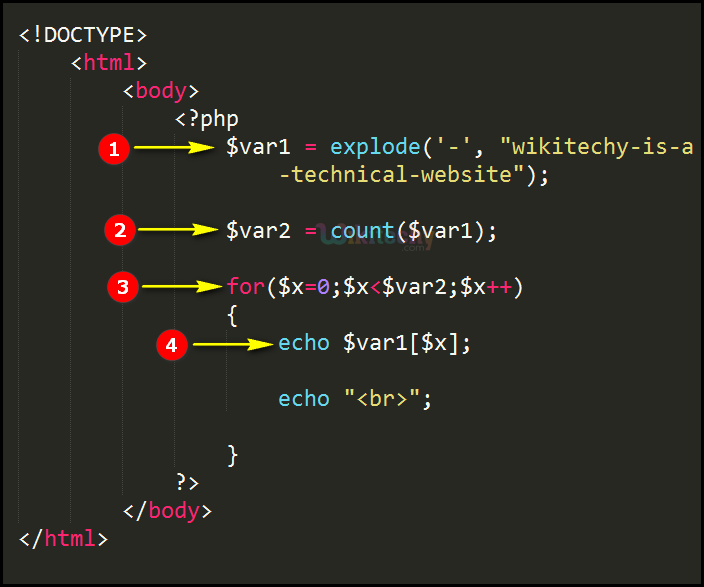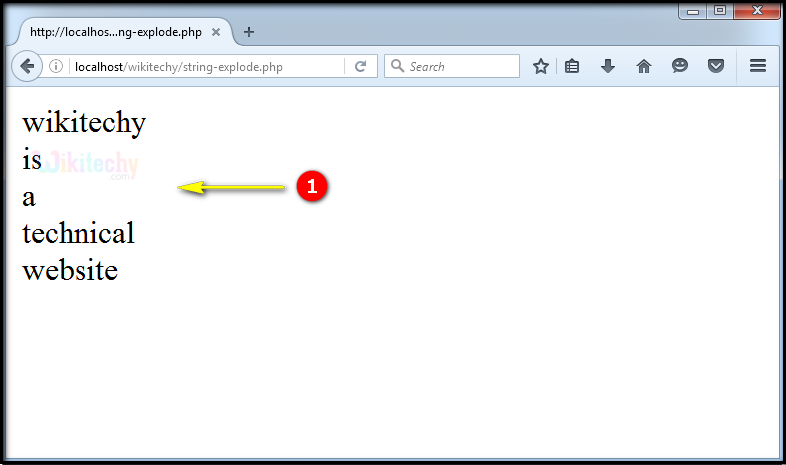# php tutorial - PHP explode Function - php programming - learn php - php code - php script

• The explode function is being used for converting strings into an array variable by separating the values.

## Syntax Explanation:

• The delimiter parameter is defining the separator operator.
• Second parameter is defining the content string.

## php for beginners Code Explanation :1. In this statement we define the value for the variable \$var1 and the explode function is used for splitting the element value according to the specified operator (Here we used the operator ‘ – ‘).
2. Variable “\$var2” is used for counting the number of values presented in the variable “\$var1”.
3. In this statement we use for loop for processing the array value.
4. Here, we display the value of the variable “\$var1” in the browser using “echo” statement.

## php coding Sample Output :1. The output statement is, “wikitechy-is-a-technical-website” the term will be splited according to the hyphen (-) and the output will be shown as above.